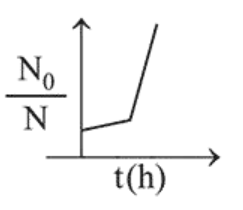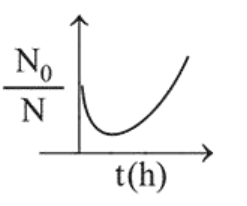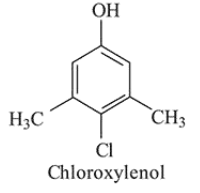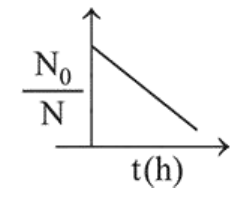# A bacterial infection in an internal wound grows as

Question:

A bacterial infection in an internal wound grows as $\mathrm{N}^{\prime}(\mathrm{t})=$ $\mathrm{N}_{0} \exp (\mathrm{t})$, where the time $\mathrm{t}$ is in hours. A dose of antibiotic, taken orally, needs 1 hour to reach the wound. Once it reaches there, the bacterial population goes down as $\frac{\mathrm{d} \mathrm{N}}{\mathrm{dt}}=-5 \mathrm{~N}^{2}$. What will be the plot of $\frac{\mathrm{N}_{0}}{\mathrm{~N}} \mathrm{vs}$. t after 1 hour?

1.2.3.4.Correct Option:

Solution:

When drug is administered bacterial growth is given by $\frac{d N}{d t}=-5 N^{2}$

On integrating the above equation,

$\Rightarrow \frac{\mathrm{N}_{0}}{\mathrm{~N}_{\mathrm{t}}}=1+5 \mathrm{t} \mathrm{N}_{0}$

The above equation is similar to straight line equation

with positive slope.

Thus $\frac{\mathrm{N}_{0}}{\mathrm{~N}_{\mathrm{t}}}$ increases linearly with $\mathrm{t}$.